# HBSE 9th Class Science Important Questions Chapter 3 Atoms and Molecules

Haryana State Board HBSE 9th Class Science Important Questions Chapter 3 Atoms and Molecules Important Questions and Answers.

## Haryana Board 9th Class Science Important Questions Chapter 3 Atoms and Molecules

Question 1.
Which two laws did the scientist Antonie L. Lavoisier establish who laid the foundation of chemical sciences?
(1) Law of Conservation of Mass.
(2) Law of Constant Proportions.

Question 2.
What is the law of conservation of mass?
According to the law of conservation of mass, during the chemical reaction mass is neither created nor destroyed.

Question 3.
What is the law of constant/definite proportions?
According to the law of constant/definite proportions, in pure chemical matter, element always exist in definite proportions by mass.

Question 4.
in accordance with mass in ammonia (NH3), what is the ratio of nitrogen and hydrogen ?
According to mass in ammonia (NH3), the ratio of nitrogen and hydrogen is 14:3.Question 5.
In water (H2O), according to mass what is the ratio of hydrogen and oxygen ?
In water (H2O), according to mass the ratio of hydrogen and oxygen is 1 : 8.

Question 6.
What is an atom ?
Atom is that smallest particle of an element, in which all the chemical properties of that element are present and it also maintains those properties.

Question 7.
Which principle did John Dalton introduce regarding the nature of matter ?
According to John Dalton, all the matter, whether element, compound or mixture are formed of small particles, which are called atoms.

Question 8.
Which are the basic particles of atom ? .
The basic particles of atom are proton, neutron and electron.

Question 9.
Which microscope is used to catch the photograph of atom ?
To catch the photograph of atoms Scanning Tunneling Microscope (STM) is used.

Question 10.
Define a molecule.
A molecule is that smallest particle of an element or a compound which has its individual or independent existence and it displays all the properties of that substance (element or compound).

Question 11.
Write down the names and symbols of any two monoatomic molecules.
Argon (Ar) and Helium (He). –

Question 12.
Write down the formulae of two diatomic and tetra-atomic molecules.
Diatomic molecules – H2, N2, HCl
Tetra-atomic molecules – H2O, CO2Question 13.
What is meant by poly-atomic molecules ?
The molecules that have more than four atoms, are called poly-atomic molecules like Sg and C2HsOH.

Question 14.
What information does a molecular formula of a substance provides us ?
The molecular formula of a substance tells us that how many atoms of every type coexist in its single molecule.

Question 15.
What is the relationship between nanometre and metre ?
1 nanometre (nm) = 10-9 metre (m).

Question 16.
What is measure unit to measure atomic radius?
Atomic radius is measured in nanometre (nm).

Question 17.
What is the atomic radius of hydrogen?
The atomic radius of hydrogen is 10-10 metre.

Question 18.
What does the abbreviation IUPAC stand for?
IUPAC means International Union of Pure and Applied Chemistry.

Question 19.
What is the symbol of iron and where does it originate from?
The symbol of iron is Fe which originates from its Latin name Ferrum.

Question 20.
Which are the Latin names from where the symbols of sodium and potassium originated ?
The symbols of sodium (Na) and potassium (K) originated from the Latin names Natrium and Kalium respectively.Question 21.
What is the difference between N2 and 2N ?
N2 is a molecule of nitrogen, whereas 2N are two atoms of nitrogen. .

Question 22.
What is meant by structural formula ?
Structural formula is a chemical formula that shows how do atoms in a molecule remain united on combined.

Question 23.
What is the structural formula of water ?
The structural formula of water is H-O-H.

Question 24.
What is the formula of carbon monoxide ?
The formula of carbon monoxide is CO.

Question 25.
Define atomic mass unit (a m u).
Atomic mass unit (a m u) is defined w.r.t. $$\frac {1}{2}$$ part mass of atom of a particular isotope of carbon (C-12).

Question 26.
What is meant by molecular mass ?
The molecular mass of a substance is the sum total of atomic mass of all the atoms existing in the one molecule of that substance.

Question 27.
What is meant by mass formula ?
The mass formula of a substance is the sum total of atomic mass of the entire atoms existing in the formula unit of that compound, whether
it is a molecule or not, it doesn’t concern.

Question 28.
What is the molecular mass of CH3OH ?
The molecular mass of CH3OH = (12 + 3 × 1 + 16 + 1)u = (12 + 3 + 16 + 1) u = 32u

Question 29.
What is the numerical unit of atoms and molecules?
The numerical unit of atoms and molecules is mole.

Question 30.
Which micro-particles combine to form molecules?
Atoms.

Question 31.
Who provided information about atom and when?
John Dalton in 1808 A.D. ‘

Question 32.
What is called as writing of an element in short form?
Symbol.

Question 33.
What is the symbol of copper ?
Cu.Question 34.
What is the symbol of aluminium ?
Al.

Question 35.
Write down the symbols of gold and silver.
Gold = An, Silver = Ag

Question 36.
What is meant by chemical formula ?
Representation of structure of a molecule of a compound through chemical symbols is called chemical formula.

Question 37.
What is meant by atomicity ?
Number of atoms present in a molecule of an element is called its atomicity.

Question 38.
What is the atomicity of inert gases?
One.

Question 39.
Write the name of a triatonic atom.
Ozone (O3).

Question 40.
What are the molecules possessing two atoms called ?
Diatomic.

Question 41.
What is the atomicity of phosphorus and sulphur ?
The atomicity of Phosphorus is four and that of sulphur is eight.

Question 42.
Give the chemical formula of copper chloride.
CuCl2.

Question 43.
What is meant by chemical equation ?
The symbolic representation of a chemical reaction is known as chemical equation.

Question 44.
What is mole ?
Mole is the quantity of matter in which 12 g of C-12 the number of molecules (atoms, molecules or ions) that have an equal number of atoms.

Question 45.
Avogadro’s constant (6.022 × 1023 mol-1) is defined as the number of atoms present in 12 grams of 12 C.

Question 46.
Define molar mass.
The mass of one-mole molecules of a substance is called as molar mass.Question 47.
What is the symbol and unit of molar mass?
Symbol: M.
Unit: g/mol or Gram per mole.

Question 48.
Give the molar mass of water and oxygen.
M(H2O) = 18 g/mol
M (O2) = 32 g/mol.

Question 49.
What are charged particles called?
Charged particles are called ions.

Question 50.
What is meant by anion and cation?
Negatively charged particles are called as anions and positively charged particles are called cations.

Question 1.
Discuss the basic principle introduced by John Dalton regarding the nature of matter.
The basic principle introduced by John Dalton regarding the nature of matter states that all substances whether they are elements, compounds or mixtures are composed of minute particles called atoms. It can be discussed as follows:
(1) Element is composed of very tiny indivisible particles, which are called atoms.
(2) All the atomic masses and characteristics or properties of a given element are equal in both. Atoms
of different elements have different masses and properties.
(3) The creation and destruction of atoms of an element cannot be transformed into atoms of other element.
(4) When atoms of different elements combine themselves in smaller but complete ratio, then they form compounds.
(5) In the given compound the relative number and kinds of atoms remain constant.
In brief, atom is the smallest particle of an element that keeps its chemical identity in all chemical and physical changes. The atoms of an element are different from the atoms of other elements.

Question 2.
How is modern concept different from that of the Daltons principle in terms of atom ?
According to Daltons opinion, atom is the smallest and indivisible particle of matter and atom can neither be created nor destroyed, but according to the modem concept an atom can be further divided into even more smaller particles – electrons, protons and neutrons. Nowadays, by splitting the nucleus of atom, atom bomb is made, which expresses the difference from the law of indestructibility of atom.

Question 3.
What were the drawbacks found in the principle of Dalton ? Clarify with examples.
Daltons principle could not explain some of the facts, which are as follows:
(1) Diamond and Graphite are formed of the same element, even then why is there so much difference in their properties ? Diamond is the hardest and the brightest thing found in nature and graphite on the other hand, is soft and of black colour.
(2) According to the principle of atom, atom is an indivisible particle, but according to the modem discoveries; atom is formed of protons, electrons and neutrons in a combined way.
(3) Mass of two atoms of an element can also be different like uranium has two isotopes 92U235 and 92U238whose atomic mass is 235u and 238u respectively.Question 4.
Explain that atom is divisible rather than indivisible.
A comprehensive study has proved that atoms of certain elements release radiations that are capable to penetrate through many substances like paper. The emission of radiations or particles from atoms proves this thing that atoms are not indivisible but, divisible.

Question 5.
How big are atoms in size?
Atoms are very small. They are even smaller than a thing that we can imagine or compare. When millions of atoms are stacked, then it would hardly form a thick layer same as thick that of a sheet of paper.

Question 6.
Show the radii of the following substances in metres:
(1) Atom of hydrogen
(2) Molecule of water
(3) Molecule of haemoglobin
(4) Grain of sand.

 Name of Substance Radii (in metres) (1) Atom of hydrogen 10-10 (2) Molecule of water 10-9 (3) Molecule of haemoglobin 10-8 (4) Grain of sand 10-4

Question 7.
Fill in the blanks:
(1) Element is composed of only one type of
(2) In a hydrogen molecule, the number of hydrogen atoms are
(3) A compound is formed of with the combination of at least elements.
(1) atoms
(2) two
(3) two.

Question 8.
Write down the symbols of the following elements : aluminium, calcium, cobalt, iodine and mercury.

 Element Symbol Aluminium Al Calcium Ca Cobalt Co Iodine I Mercury Hg

Question 9.
Which elements do the following symbols represent ? Na, K, P, Pb, Ca, Zn, Br and Sn.

 Symbol Element 1. Na Sodium 2. K Potassium 3. P Phosphorus 4. Pb Lead 5. Ca Calcium 6. Zn Zinc 7. Br Bromine 8. Sn Tin

Question 10.
Match the statements given in Column A with the symbols given in Column B:

 Column A Column B (i) One molecule of nitrogen Na (ii) A molecule of a compound N (iii) An atom of a metal N2 (iv) One atom of nitrogen NH3

 Column A Column B (i) One molecule of nitrogen N2 (ii) A molecule of a compound NH2 (iii) An atom of a metal Na (iv) One atom of nitrogen N

Question 11.
On the basis of Latin names, write down names and symbol of some elements.

 Element Latin Name Symbol Sodium Natrium Na Copper Cuprum Cu Iron Ferrum Fe Potassium Kalium K Silver Argentum Ag Tin Stannum Sn Gold Aurum Au Mercury Hydragirum Hg Lead Plumbum Pb

Question 12.
Write down the names of the elements present in the following compounds:
Calcium Oxide (CaO), sulphur dioxide (SO2), potassium nitrate (KNO3), mercuric oxide (HgO) and borax (Na2B4O7).
Elements present in the given compounds are shown in the following chart:

 Compound Existing Element 1. Calcium Oxide (CaO) Calcium (Ca); Oxygen (O) 2. Sulphur Dioxide (SO2) Sulphur (S); Oxygen (O) 3. Potassium Nitrate (KNO3) Potassium (K);Nitrogen(N); Oxygen(O) 4. Mercuric Oxide (HgO) Mercury (Hg); Oxygen (O) 5. Borax (Na2B4O7) Sodium (Na); Boron (B); Oxygen (O)

Question 13.
Why da we not take 1/6 part of the mass of oxygen atom in the form of a unit ?
We do not take 1/6 part of the mass of oxygen atom in the form of a unit because:
(1) Oxygen forms compound after reacting with many other elements.
(2) With this atomic mass unit, the atomic masses of most of the elements are obtained in maximum number.Question 14.
How do atoms remain in existence ?
Atoms of most of the elements cannot remain in existence in independent state. Atoms form molecules and ions. These molecules or ions combine together in a huge number to form a fluid which we can see, feel or touch.

Question 15.
Show the following compounds by means of molecular models:
(i) Water
(ii) ammonia
(iii) ethyl alcohol
(iv) chlorine
(v) phosphorus
(vi) sulphur.
The molecular models of the given simple compounds are as follows: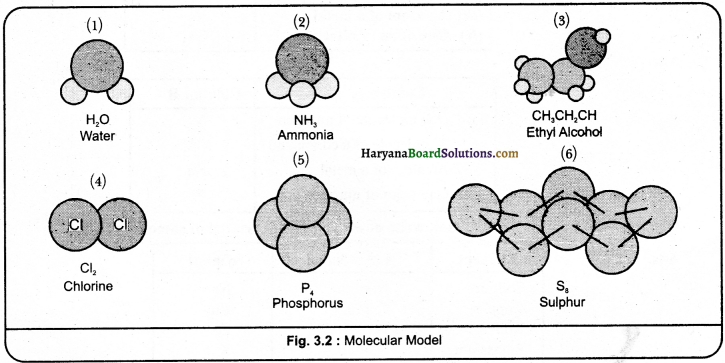Question 16.
Give the difference between the following :
(i) Hydrogen atoms and its molecules.
(ii) Molecules of oxygen and molecules of water.
(iii) Molecules of sugar and molecules of water.
(iv) Molecules of calcium oxide and molecules of magnesium oxide.
(i) The smallest unit of hydrogen (H), is the atom of hydrogen, but a molecule of hydrogen is formed of with the combination of two atoms (H2).
(ii) A molecule of oxygen consists of two atoms of oxygen (O2), but in a molecule of water there are two atoms of hydrogen and a single atom of oxygen (H2O).
(iii) A single molecule of sugar consists of 12 atoms of carbon, 22 atoms of hydrogen and 11 atoms of oxygen (C12H22O11), but a single molecule of water consists of two atoms of hydrogen and one atom of oxygen (H2O).
(iv) A single molecule of calcium oxide consists of one atom of calcium and one atom of oxygen (CaO), but in a single molecule of magnesium oxide consists of one atom of magnesium and one atom of oxygen (MgO).Question 17.
Write down the names of the elements present in the following compounds and also give the number of atoms present in the atoms:
Baking Soda (NaHCO3), Sodium Carbonate (Na2CO3), Copper Sulphate (CuSO2), Sand (SiO2), Aluminium Chloride (AlCl3):
The names of the elements present in the given compounds and the number of atoms present in each element is given in the table as given ahead:

 Compound Present Element Number of atoms (1) Baking Soda (NaHCO3) Sodium (Na) Hydrogen (H) Carbon (C) Oxygen (O) 1 1 1 3 (2) Sodium Carbonate (Na2CO3) Sodium (Na) Carbon (C) Oxygen (O) 2 1 3 (3) Copper Sulphate (CuSO4) Copper (Cu) Sulphur (S) Oxygen (O) 1 1 4 (4) Sand (Sb2) Silicon (Si) Oxygen (O) 1 2 (5) Aluminium Chloride (Aid3) Aluminium (Al) Chlorine (Cl) 1 3

Question 18.
What is meant by monoatomic, diatomic and polyatomic?
Monoatomic: Elements that are found in the form of single atom are called monoatomic. For example, Helium (He) and Neon (Ne).
2. Diatomic: Those elements that have two atoms in a single molecule, are called diatomic. For
example, Hydrogen (H2), Oxygen (O2), and Nitrogen (N2).
3. Polyatomic: The elements that consist of more than two atoms in their single molecule are called
polyatomic. For example, Phosphorus (P4) , and Sulphur (S9).

Question 19.
What is meant by atomicity? Write down formulae and atomlcity 01 some elements.
The number of atoms present in a molecule of an element is called its atomicity. The formulae and atomicity of some elements is as follows:

 Element Formula Atomicity Hydrogen H2 2 Helium He 1 Nitrogen N2 2 Oxygen O2 2 Neon Ne 1 Phosphorus P4 4 Sulphur S8 8 Chlorine Cl2 2 Bromine Br2 2

Question 20.
What things should be kept in mind while writing a chemical formula of a compound ?
While writing a chemical formula of a compound, following things should be kept in mind:
(1) The valency or change of ion should be balanced.
(2) When a compound is made up of any metal or non-metal, then the name of the metal or its symbol is written in chemical formula before. For example, Calcium Oxide (CaO), Sodium Chloride (NaCl), Iron Sulphide (FeS), Copper Oxide (CuO), etc. where oxygen, chlorine, sulphur are non-metals and they are written on right hand side, whereas calcium, sodium, iron and copper are metals and they are written on left nand side.
(3) In compounds composed of polyatomic ions, ions are put into brackets first, after that the number showing the proportionalities is written.Question 21.
The chemical formula of water is H2O. What information do we get from it ?
We get the following information from the chemical formula of water H2O:
(1) We come to know from the chemical formula that the given compound H20 is formed of the combination of hydrogen and oxygen.
(2) Also, we come to know about the number of atoms of each element present in the single molecule of a substance through the chemical formula i.e., there are two atoms of hydrogen and one atom of oxygen in H2O.
(3) Through chemical formula, the valency of each element present in a given substance is understood or in H2O, the valency of oxygen is 2 and that of hydrogen is 1.
(4) Through chemical formula, we come to know the molecular mass of the given substance i.e., the molecular mass of H2O is 1 x 2 + 16 = 18 u.
(5) Through chemical formula we come to know that what the proportion of the number of atoms of
the components of elements is and the ratio from the point of view of mass in the given substance. i’. e., in H2O, H: O = 2 : 1 and from the point of view of mass H : O = 2 : 16 or = l : 8.
(6) The chemical formula shows that the given compound is electrically neutral i. e. H2O does not possess any charge on it.

Question 22.
How is molecular mass or molecular weight calculated with the help of formula?
In the following way the molecular mass or molecular weight is calculated :
(1) First of all write down the chemical formula of the compound.
(2) Now write down each element separately.
(3) Then write down the number of atoms of all elements.
(4) Thereafter, write down the atomic mass of the elements.
(5) Now, multiple the number of atoms of the elements with their atomic masses.
(6) Now add all the products. This is the real mass of the compound.
For Example:

 Formula Element Number of atom Number of atom Atomic mass Molecular mass H2O (Water) H O 2 1 2 1 1 16 2 x 1 = 2 1 x 16 = 16 Molecular mass = 18

Question 23.
How many moles of chlorine atom are there in each mole of the following compounds?
(a) Cl2O7
(b) HCl
(e) BaCl2
(d) AlCl3
(e) FeCl3
(f) CCl4
Solution:

 Compound Moles of chlorine atom (a) Cl2O7 2 (b) HCl 1 (e) BaCl2 2 (d) AlCl3 3 (e) FeCl3 3 (f) CCl4 4

Question 24.
How is the mass percentage of element Aand element B in the given compound AB calculated?
AB is a compound whose one element is A and the other element is B. We give the mass percentage of A in terms of AB of its one hundred parts in the form of division of A.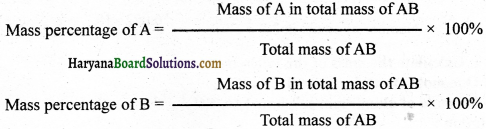Numerical Questions

Question 1.
Calculate the molecular mass of the following compounds:
(i) NH3
(ii) NaCl
(iii) (NH4)2 SO4
(iv) Al2 (SO4)3
(v) C6H12O6
(vi) CaCO3
[N = 14, H 1, Na 23, Cl = 35.5, S = 32,0 16, Al = 27, C = 12, Ca = 401]
Solution:
(1) Molecular mass of NH3 = [14 + 3 × 1] u
= [14 + 3]u
= 17u

(ii) Molecular mass of NaCI = [23 + 35.5] u = 58.5 u

(iii) Molecular mass of (NH4)2 SO4 = [2(14 + 4 × 1) + 32 + 4 × 16] u
= [2(18) + 32 + 64]u
= [36 + 32 + 64]u
= 132u(iv) Molecular mass of A12(S04)3 = [2 × 27 + 3 (32 +4 × 16)] u
= [54 + 3(32 + 64)lu
= [54 + 3(96)]u
= [54 + 288]u
= 342u

(v) Molecular mass of C6H12O6 [6 × 12 + 12 × + 6 × 16] u = [72 + 12 + 96] u = 180u

(vi) Molecular mass of CaCO3 [40 + 12 + 3 x 16] u
= j40+12+48]u
= 100 u

Question 2.
Find number of molecules in the following:
(i) 0.25 mole of oxygen gas
(ii) 66 g carbon dioxide
Solution:

(i) Number of molecules in I mole oxygen = 6.022 × 1023 molecules
∴ Number of molecules in 0.25 mole oxygen = 6.022 × 1023 × 0.25 molecules = 1.505 × 1023 molecules

(ii) We know that I mole carbon dioxide (CO2) = = (1 × 12 + 2 × 16]g = (12 + 32)g = 44g
Number of molecules in 44g carbon dioxide = 6.022 × 1023 molecules
∴ Number of molecules in 66g carbon dioxide = $$\frac{6.022 \times 10^{23}}{44}$$ × 66 molecules
= 9.033 × 1023 molecules

Question 3.
How many atoms of iron will be there in the piece of iron (Fe) with 2.8 gram mass ?(Fe = 56g)
Solution:
One mole of atoms of iron = 6.022 × 1023 atom = 56g
Number of atoms in 56 g of iron = 6.022 × 1o23
Number of atoms in 2.8 g of iron = $$\frac{6.022 \times 10^{23}}{56}$$ × 2.8 = 3.011 × 1022 atoms

Question 4.
Calculate the mass of the following in grams:
(a) One mole of sulphuric acid (H2SO4),
(b) 0.5 mole of aluminium sulphate (Al2(SO4)3).
Solution:
(a) Mass of one mole of H2SO4 in grams = 1 × 2 +32 + 16 × 4
= 2 + 32 + 64
= 98g

(b) Mass of one mole of Al2 (SO4)3 in grams = 27 × 2 + 3(32 + 16 × 4)
= 54 + 3(32 + 64)
= 54 + 3 × 96
= 54 + 288 = 342g
= 54 + 288 = 342g
∴ Mass of 0.5 mole of Al2(SO4)3 in grams = 342 × 0.5
= 342 × $$\frac {5}{10}$$ = 171 g

Question 5.
Calculate the number of moles of CaCO3 of 20 g of calcium carbonate (CaCO3).
Solution:
Onemole of CaCO3 = 40 × 1 + 12 × 1 + 16 × 3
= 40 + 12 + 48
= 100g

Question 6.
In aluminium sulphate Al2(SO4)3 of 34.2 g salt, how many moles of each element will be there?
Solution:
One mole of Al2(SO4)3 = 2 × 27 + 3 × 32 + 12 × 16 = 54 + 96 + 192 = 342g
(1) Number of Al moles in 342 g of Al2 (SO4)3= 2 mole
∴ Number of Al moles in 34.2 g of Al2 (SO4)3 = $$\frac{2}{342}$$ × 34.2 = 0.2 mole

(2) Number of S moles in 342 g of Al2 (SO4)3 = 3 mole
∴Number of S moles in 34.2 g of Al2 (SO4)2 = $$\frac{3}{342}$$ × 34.2 = 0.3 mole

(3) Number of O moles in 342 g of Al2 (SO4)3 = 12 mole
∴Number of O moles in 34.2 g of Al2 (SO4)3 = $$\frac{12}{342}$$ × 342 = 1.2 mole

Question 7.
How many molecules of water are there in 1 g of H2O?
Solution:
I mole of H2O = 1 × 2 + 16 × 1
= 2 + 1618g
∴Molecules in 18 g (one mole) H2O = 6.022 × 1023
Molecules in 1 g H2O = $$\frac{6.022 \times 10^{23}}{18}$$
= 3.346 × 1022Question 8.
Convert the following into moles:
(i) 3.012 x 1023 Sodium atoms
(ii) 180 g water
Solution:
(i) 6.022 × 1023 Sodium atoms 1 mole sodium
∴3.0 12 × 1023 Sodium atoms = 6.022x 1023 × 3.012 × 1023 mole sodium

(ii) We know that 1 mole water (H2O) (2 × 1 + 1 × 16] g = 2 + 16 = 18g
∵ 18 g water = 1 mole water
∴180 g water = $$\frac {1}{18}$$ × 180 mole water = 10 mole water

Question 9.
Determine the mass of 6.022 × 1023 N2 molecules.
Solution:
1 mole N2 = 2 × 14 = 28g
= 6.022 × 1023 molecules
∴Mass of molecules 6.022 x 1023 molecules N2 = 28g

Question 10.
Calculate the molar mass of the following and write down their suitable standard units.
C2H5OH, S8, PCl5
Solution:
We know that mass of one mole of a substance is called as its molar mass.
(i) Molar mass of C2H5OH = (2 x 12 + 5 × 1 + 16 + 1)g/mol
= (24 + 5 + 16 + 1) g/mol = 46 g/mol

(ii) Molar mass of S8 = (8 x 32 = 256) g/mol
(iii) Molar mass of PCl5 = (3 1 + 5 × 35.5) g/mol = (3 1 + 177.5) g/mol
= 208.5g/mol

Question 11.
Calculate the number of particles in 0.1 mole carbon atom.
Solution:
1 mole carbon = 6.022 × 1023 particles
0.1 mole carbon = 0.1 × 6.022 × 1023 particles = 6.022 × 1022 particles

Question 12.
Calculate the molecular mass of the following:
(i) PCl5
(ii) NH3
(iii) CH2 Cl2
(iv) H2O2
(v) S8
(vi) HCl
Where-P=31, Cl=35.5, N=14, H=1, Cl2, O16, S=32
Solution:
(i) Molecular mass of PCl5 = [31 + 5 x 35.5] u = [31 + 177.5] u = 208.5 u
(ii) Molecular mass of NH3 = [14 + 3 × l]u = 17u
(iii) Molecular mass of CH2Cl2 = [12 + 2 × 1 + 2 × 35.5] u = [12 + 2 + 71]u = 8Su
(iv) Molecular mass Of H2O2 = [2 x 1 + 216]u = [2 + 32] u = 34u
(v) Molecular mass of S8 = 8 x 32u = 256u
(vi) Molecular mass of HCl = [1 + 35.5] u = 36.5 uQuestion 13.
Calculate the mass formula of the following compounds:
(i) MgO
(ii) CaCl2
(iii) CaCO3
(iv) MCl3
Solution:
(i) Mass formula of MgO = [24 + 16] g = 40 g
(ii) Mass formula of CaCl2 = [40 + 2 x 35.5] g = [40 + 71]g = lllg
(iii) Mass formula of CaCO3 = [40 + 12 + 3 x 16] g = [40 + 12 + 48] g = 100 g
(iv) Mass formula of Al Cl3 = [27 + 3 x 35.5] g = 127 + 106.51g = 133.5g

Question 14.
Calculate the percentage of each element in I mole formaldehyde (CH2O).
Solution:
Molar mass of CH2O [12 + 2 x 1 + 16] g/mol = 3Ogfmol
Percentage of C in CH2O = $$\frac {π}{2}$$ x 100 = 40%
Percentage of H in CH2O = $$\frac{2 \times 1}{30}$$ x 100 = 6.7%
Percentage of O in CH2O = $$\frac{16}{30}$$ x 100 = 53.3%

Question 15.
How many atoms of each element are there in 6.3 g of nitric acid (HNO3)?
Solution:
Molecular mass of HNO3 = 1 x 1 + 14 x 1 + 16 x 3 = 1 + 14 + 48 = 63 u
Mass of I mole of HNO3 = 63 g
63g HNO3 = 1 mole
∴63g HNO3 = $$\frac{1 \times 6.3}{63}$$ mole = 0.1 mole
(1) Number of H-atoms in I mole HNO3 = 1 mole H – atoms
= 6.022 x 1023 H-atoms (Avogadro’s number)
∴Number of H-atoms in 0.1 mole HNO3 = $$\frac{6.022 \times 10^{23} \times 0.1}{1}$$
= 6.022 x 1023 H-atoms

(2) Number of N-atoms in I mole HNO3 = 1 mole N-atoms
= 6.022 x 1023 N-atoms
∴Number of N-atoms in 0.1 mole HNO3 = $$\frac{6.022 \times 10^{23} \times 0.1}{1}$$
= 6.022 x 1022 N-atoms

(3) Number of 0-atoms in I mole HNO3 = 3 mole 0-atoms
= 3 x 6.022 x 1023 0-atoms
= 18.066 x 1022 0-atoms
Number of 0-atoms in 0.1 mole HNO3 = $$\frac{6.022 \times 10^{23} \times 0.1}{1}$$
18.066 x 1o 0-atoms Ans

Question 16.
Calculate number of chloride ions in 9.Sg of magnesium chloride.
(Atomic mass: Mg = 24, Cl = 35.5)
Solution:
1 mole of magnesium chloride (MECl2) = mass of Mg + mass of Cl x 2 = 24 + 35.5 x 2 = 24 + 71 = 95g
∵ 95g Magnesium Chloride = 1 mole
∴ 9.5g Magnesium Chloride = $$\frac {1}{95}$$ x 9.5 mole = 0.1 mole
∵ 1 moIe Magnesium Chloride = 2 x 6.022 x 1023 Chloride ions
∴ 0.1 mole Magnesium Chloride = 0.1 x 2 x 6.022 x 1023 Chloride ions
= 1.2044 x 1023 Chloide ionsQuestion 17.
Calculate Molecular mass of the following compounds.
Na2SO4, ZnCO3, CaSO4, H2SO3, HNO2 and N2O5
(Atomic Mass): (Na 23.04, S = 32.04, 0 = 16.04, Zn = 65.04, C = 12.04, Ca = 40.04, 11 = 1.04, N= 14.04)
(i) Molecular mass of Na2SO4 = [2 x 23 + 1 x 32 + 4 x 16] u = [46 + 32 + 64]u = 142u
(ii) Molecular mass of ZnCO3 [1 x 65 + 1 x 12 + 3 x 16] u = [65 + 12 + 48]u = 125u
(iii) Molecular mass of CaSO4 = [1 x 40 + 1 x 32 + 4 x 16] u = [40+ 32+ 64] u = 136u
(iv) Molecular mass of H2SO3 = [2 x 1 + 1 x 32 + 3 x 16]u = [2 + 32 + 48]u = 82u
(v) Molecular mass ofHNO2 = [1 x 1 + 1 x 14 + 2 x 16]u = [1 + 14 + 32]u = 47u
(vi) Molecular mass of N2O5 = [2 x 14 + 5 x 16] u = [28 + 80] u = 108u

Do Yourself

Question 1.
Calculate the number of moles in the following:
(i) 52g helium (get mole from mass).
(ii) 5.6g Fe
(i) 13 mole
(ii) 0.1 mole.

Question 2.
Non-gas has single atoms. In how much rass of neon will there be 6.022 x 1023 atoms?
20g

Question 3.
(i) In 9 g water
(ii) In 17 g ammonia how many molecules will be present?
(i) 3.011 x molecules
(ii) 6.022 x 1023 molecules.

Question 4.
Find out the number of moles in 17g H2O2
0.5 mole.

Question 5.
Calculate the masses of the follòwing:
(i) 0.5 mole N2 gas (mass from the mole of molecule).
(ii) 0.5 mole N atoms (mass from the mole of atom).
(iii) 3.011 x 1023N number of atoms (mass from number).
(i) 142
(ii) 72
(iii) 72.Question 6.
Calculate the molar mass of the following substances:
(i) Ethyne (C2H2)
(ii) Molecular Sulphur (S8)
(iii) Phosphorus molecule (P)
(iv) Hydrochloric acid (HCl)
(v) Nitric acid (HNO3)
(i) 26g/mol
(ii) 256g/mol
(iii) 124 g/mol
(iv) 36.5g/mol
(v) 63g/mol.

Question 7.
What is the mass of the following?
(i) 0.2 moles atoms of oxygen.
(ii) 0.5 moles molecules of water
(i) 3.2g.
(ii) 9g

Question 8.
Calculate the number of particles in each of the following:
(i) 46g Sodium atom (number from mass).
(ii) 8g Oxygen molecule (number of molecules from mass).
(i) 12.044 x 1023
(ii) 1.51 x 1023

Question 9.
(i) Calculate the number of Sulphur (S8) molecules In 16 g of solid sulphur.
(ii) Calculate the number of ions of Aluminium In 0.056 g of Aluminium Oxide (Al2O3).
(i) 3.76 x 1022 molecules
(ii) 6.612 x 1020 ions.

Question 10.
Calculate the percentage of each element en 1 mole ethyl alcohol (C2H5OH).
C 52.17%, H 13.04%, 0 34.79%.

Question 11.
Calculate the percentage of oxygen In the following compounds:
(i) HgO
(ii) K2CrO7
(iii) Al2
(iv) (SO)3
(i) 7.39%
(ii) 37.84%
(ii,) 56.14%.

Question 12.
What will be the mass in grams of the following compounds?
(i) 0.40 mole CO2
(ii) 3.00 mole NH3 mole
(iii) 5.14 mole H5IO6
(i) 17.6 g
(ii) 512 g
(iii) 1171.92 g

Question 13.
A compound ‘X’ after analysing produces 85.72% carbon and 14.28% hydrogen. 1f the molar mass of the compound is 28, then find out its molecular formula.
Solution : Proportionality formula = C2H4Essay Type Questions

Question 1.
Explain the method to find the molecular formula of a compound.
By getting the following significant knowledge about an unknown compound, the molecular formula of the compound can be known:
(1) First of all, percent number of compounds should be known. For that, find out the percentage of different elements in the compound.
(2) Find out the atomic mass of elements in the Periodic Table.
(3) Find out the atomic ratio of elements by dividing per cent quantity of elements by atomic mass.
(4) To findthe simplest ratio of elements divide the ratio of elements by the least ratios among those.
(5) Write the symbols of elements side by side. On the right-hand side below them, write down the ratio of the concerned atom. Thus, the basic proportionality of the formula will be known.
(6) By writing the mass of elements in the basic proportionality formula, find out the mass of basic proportionality formula.
(7) Find out the proportionality number ‘n’ by dividing molecular mass with .baic proportionality mass for Thula.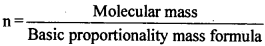(8) By multiplying basic proportionality formula with n molecular formula can be known Molecular formula basic proportionality formula xn.

Question 2.
Write down the formulae of the following compounds: .
(1) Hydrogen Chloride
(2) Hydrogen Sulphide
(3) Carbon tetrachloride
(4) Magnesium Chloride
(5) Calcium Oxide
(6) Aluminium Oxide.
While writing the chemical formulae of molecular compounds, the symbols of each formed combined elements are written, thereafter, their valencies are written. Finally, the valencies of the combined atoms are crossed over and then the molecular formula is written.
(1) Formula of Hydrogen Chloride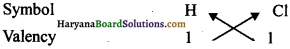∴ Formula of Hydrogen Chloride = HCl

(2) Formula of Hydrogen Sulphide∴Formula of Hydrogen Suiphide H2S

(3) Formula of Carbon tetrachioride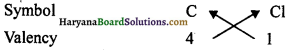∴Formula oTCarbon tetrachlonde = CCl4

(4) Formula of Magnesium Chloride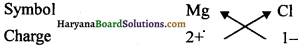∴Formula of Magnesium Chloride = MgCl2

(5) Formula of Calcium Oxide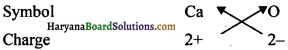Here the valences of both elements is same. Hence, its formula will be Ca2O2, but we write this formula in a simple form i.e., CaO.

(6) Formula of Aluminium Oxide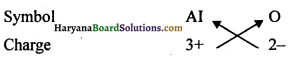∴Formula of Aluminium Oxide = Al2O3Question 3. Write down formulae of the compounds of the following: .
(i) Sodium nitrate
(ii) Calcium hydroxide
(iii) Ammonium sulphate
(iv) Sodium carbonate.
(i) Formula of Sodium Nitrate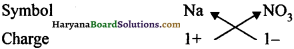∴ Formula = NaNO3
(ii) Formula of Calcium Hydroxide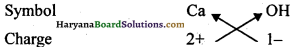∴ Formula Ca(OH)2

(iii) Formula of Ammonium Sulphate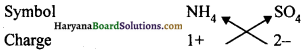∴Formula = (NH4)2SO4

(iv) Formula of Sodium Carbonate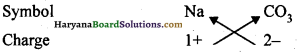∴Formula = Na2CO3

Practical Work

Experiment 1.
Prove with an experiment the law of solutions conservation of mass.

Procedure:
Prepare a 5% solution of copper sulphate and sodium carbonate in water. Now, take a little quantity of sodium carbonate solution in a conical flask and take a little quantity of copper sulphate in a test tube and suspend it into the conical flask in such a way that both the solvates should not get mixed up with each other as shown in the figure. After that fix it to the neck of flask and find its mass. Now, rotate the flask in a tilted position so that both the solutions get mixed up together. Now, again note down the mass of the flask. You will notice that before and after the activity the mass of the conical flask remains constant. This proves the law of mass conservation.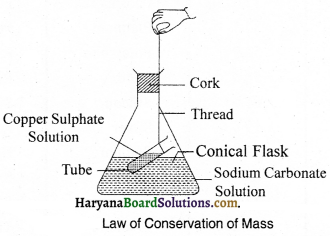Experiment 2.
Describe an activity to explain the standard atomic mass unit.

Procedure:
Suppose a fruit seller is selling fruits without standard weights. He takes a watermelon and says “This has a mass equal to 12 units.” He chops the watermelon into 12 equal pieces and finds that the mass of each fruit being sold by him is relative to the mass of the single piece of the watermelon. As shown in the figure. Now, he sells the fruits by relative fruit mass unit (frnu). Thus, the relative atomic mass of an element is defined as average mass of its atoms w.r.f. the mass of 1/12 th part the mass of one carbon-l2 atoms.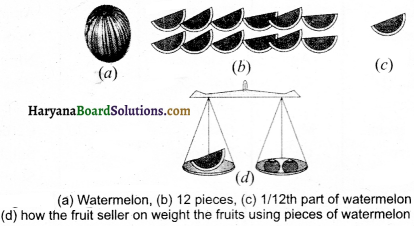Important Information And Tables

List of Symbols and Atomic Masses of Some Common Elements

 Element Symbol Mass (u) Aluminium Al 27.0 Argon Ar 39.0 Arsenic As 74.9 Barium Ba 137.3 Boron B 10.8 Bromine Br 79.9 Calcium Ca 40.1 Carbon C 12.0 Chlorine Cl 35.5 Chromium Cr 52.9 Cobalt Co 58.9 Copper Cu 63.9 Fluorine F 19.0 Gold Au 197.0 Helium He 4.0 Hydrogen H 1.008 Iodine I 126.90 Iron Fe 55.8 Lead Pb 207.2 Lithium Li 207.2 Xenon Xe 131.3 Magnesium Mg 24.3 Manganese Mn 54.9 Mercury Hg 200.6 Neon Ne 20.1 Nickel Ni 58.7 Nitrogen N 14.0 Oxygen 0 16.0 Phosphorus P 31.0 Platinum Pt 195.1 Potassium K 39.1 Radon Rn 222 Silicon Si 28.1 Silver Ag 107.9 Sodium Na 23.0 Sulphur S 32.1 Tin Sn 118.7 Titan j urn Ti 47.9 Tungsten W 183.8 Uranium U 238.0 Vanadium V 50.0 Zinc Zn 65.4

Some Common, Simple and Polyatomic ions

 Valency Name of ion Symbol Non-metallic Symbol Polyatomic ions Symbol 1 Sodium Na+ Hydrogen H+ Ammonium NH4+ 1 Potassium K+ Hydride H– Hydroxide OH– Silver Ag+ Chloride Cl– Nitrate NO3– 1 Copper (I)* Cu+ Bromide Br– Hydrogen 2 Magnesium Mg2+ Iodide I– Carbonate HCO32- 2 Calcium Ca2+ Oxide O2- Carbonate CO32- 2 Zinc Zn2+ Sulphide S2- Sulphite SO32- 2 Iron (II)* Fe2+ Sulphate SO42- 2 Copper (II)* Cu2+ 3 Aluminium Al3+ 3 Iron (III)* Fe3+ Nitride N3- Phosphate PO43-

Quick Review of the Chapter

1. Which Indian scientist gave name of atom to smallest indivisible particle of an element?
(B) Pakudha
(D) Dr. Raman

2. Who proposed the law of conservation of mass?
(A) Democratis
(B) Leucippus
(C) Lavoisier and Joseph L. Proest
(D) John Dalton
(C) Lavoisier and Joseph L. Proest3. Which Indian scientist gave name of atom to smallest indivisible particle of an element?
(B) Pakudha
(D) Dr. Raman
(B) Pakudha

4. The atomic principle of John Dalton is:
(A) All liquids are composed of atoms
(B) Atoms are smallest indivisible particles
(C) The properties of atoms of an element
(D) All above mentioned
(D) All above mentioned

5. The symbol of hydrogen proposed by Dalton was: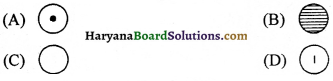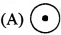6. According to Dalton, the symbol of phosphorus was: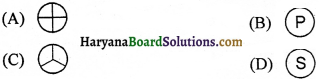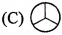7. According to tUPAC, the symbol of cobalt is:
(A) K
(B) Co
(C) CO
(D) C
(B) Co

8. According to IUPAC, the symbol of aluminium is:
(A) AL
(B) AL
(C) AL
(D) AI
(D) Al

9. According to IUPAC, the symbol of silicon is:
(A) si
(B) SI
(C) Si
(D) Cl
(C) Si10. The atomic mass of hydrogen is:
(A) 1
(B) 2
(C) 4
(D) 6
(A) 1

11. What ¡s the atomic mass of carbon?
(A) 4
(B) 6
(C) 12
(D) 16
(C) 12

12. The number of atoms of oxygen present in Ozone is:
(A) 2
(B) 3
(C) 4
(D) 5
(B) 3

13. What is the atom icity of sulphur?
(A) 8
(B) 7
(C) 5
(D) 3
(A) 8

14. What is the atomic mass of sodium?
(A) 11
(B) 23
(C) 46
(D) none of these
(B) 23

15. The atomic mass of chlorine is:
(A) 17
(B) 35
(C) 35.5
(D) 34
(C) 35.5

16. What is the mass proportion of hydroge4n and oxygen in water?
(A) 1:4
(B) 1:8
(C) 8:1
(D) 1:16
(B) 1:817. The mass proportion of nitrogen and hydrogen in ammonia is:
(A) 7:1
(B) 14:1
(C) 14:3
(D) 7:14
(C) 14:3

18. The mass proportion of carbon and oxygen in carbon-dioxide is:
(A) 3:8
(B) 16:3
(C) 8:3
(D) 2:7
(A) 3:8

19. The correct symbol of aluminium ion is:
(A) Al
(B) Al
(C) Al2
(D) Al3
(D) Al3

20. What is the Latin name of iron element?
(A) Ferum
(B) Cuprum
(C) Kalium
(D) Natrium
(A) Ferum

21. What is the chemical formula of hydrogen chloride?
(A) HCl
(B) HCl2
(C) HCl3
(D) H2Cl
(A) HCl

22. The chemical formula of hydrogen suiphide is:
(A) HS
(B) H2S
(C) H2S2
(D) H2S3
(B) H2S

23. What ¡s the formula of carbon tetrachioride?
(A) C4Cl
(B) CCl
(C) CCl4
(D) C4Cl4
(C) CCl4

24. What is the formula of sodium carbonate is:
(A) NaCO3
(B) NaCO2
(C) Na2CO3
(D) Na3CO3
(C) Na2CO325. The chemical formula of common salt is:
(A) NaCl
(B) MgCl
(C) CaCl2
(D) Ca(OH)2
(A) NaCI

26. The molecular mass HNO3 is:
(A) 53u
(B) 63u
(C) 73u
(D) 58.5 u
(B) 63 u

27. What is the molecular mass of NaCl?
(A) 36.5 u
(B) 35.5 u
(C) 58.5 u
(D) 63 u
(C) 58.5 u

28. What is the molecular mass of CaCl2?
(A) 98.2u
(B) 111u
(C) 112u
(D) 114.2 u
(B) IIlu

29. The molar mass of MCl is:
(A) 35.5 g/mol
(B) 36.5 g/mot
(C) 63 g/mol
(D) 73 g/mol
(B) 36.5 g/mol

30. The motar mass of HNO3 is:
(A) 63 g/mol
(B) 635 g/mol
(C) 26 g/mol
(D) 124 g/mol
(A) 63 g/mol

31. The symbol of potassium is:
(A) Pt
(B) K
(C) P
(D) Pm